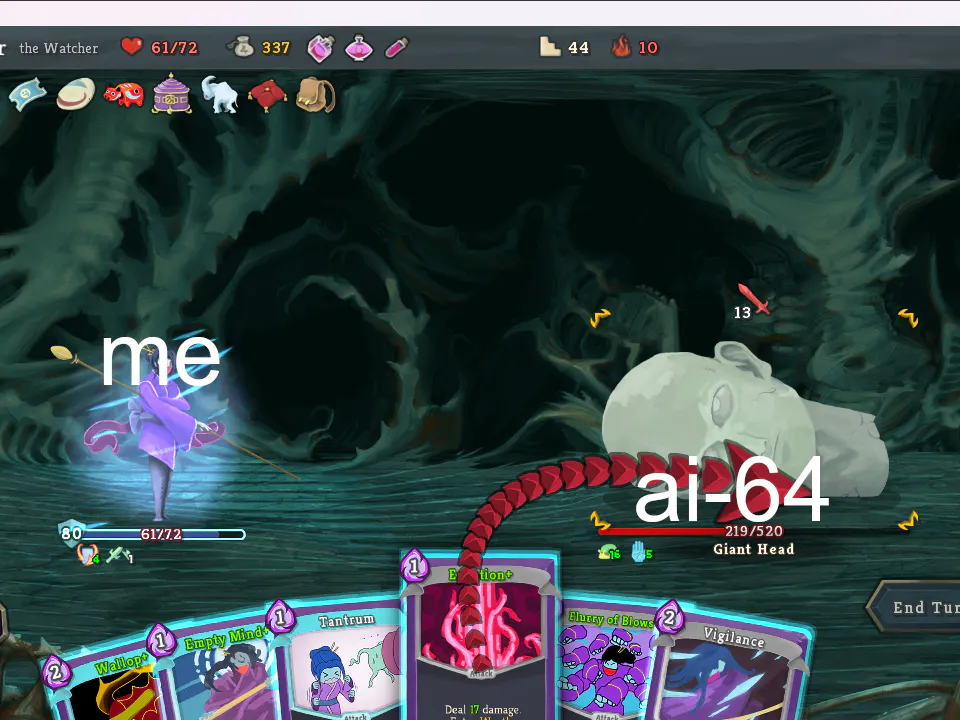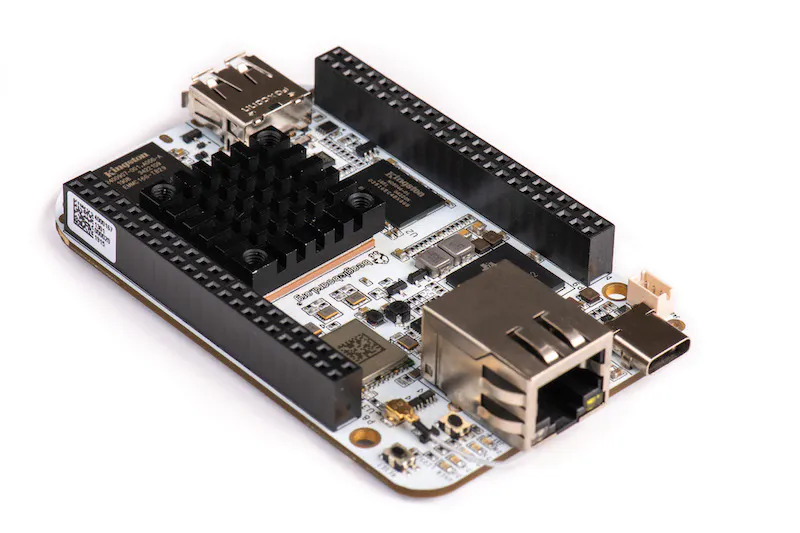Published

# Team Housekeepers' ford reptomancer

This project is a simple self-driving toy vehicle that drives along a path marked with blue painter's tape, stopping at 2 red stop markers.

IntermediateFull instructions provided10## Things used in this project

### Hardware componentsWebcam, Logitech® HD Pro
×1BeagleBoard.org BeagleBone AI
×1
×1
 portable charger
×1

### Software apps and online servicesOpenCV

## Code

### Speed encoder driver

C/C++
The driver code for the speed encoder. Treats the speed encoder as a button and reports how much time has passed since the last HIGH output from the speed encoder.
```#include <linux/gpio/consumer.h>
#include <linux/interrupt.h>
#include <linux/kernel.h>
#include <linux/module.h>
#include <linux/of_device.h>
#include <linux/platform_device.h>
#include <linux/fs.h>
#include <linux/device.h>
#include <linux/uaccess.h>
#include <linux/ktime.h>
#include <linux/timekeeping.h>

static int majorNumber;
static struct class* mescharClass = NULL;
static struct device* mescharDevice = NULL;

static int device_open(struct inode *, struct file *);
static ssize_t device_read(struct file *, char __user *, size_t, loff_t *);

static struct file_operations fops = {
.open = device_open,
};

static u64 prev_time = 0;
static u64 interval = 123;

// Matches `compatible` property in the device tree.
#define DEVICE_NAME "hello"
#define CLASS_NAME "killingai64"

// Speed encoder acts like a button.
static unsigned int irq_num;

// Handles char device open event. Nothing special.
static int device_open(struct inode *inodep, struct file *filep) {
// Debug info.
printk(KERN_INFO "You're tearing me apart, Lisa!\n");
return 0;
}

/**
* Handles char device read event. Returns the time duration in ns
* since the last "button down" event.
*/
static ssize_t device_read(struct file *filep, char __user *buf, size_t length, loff_t *offset) {
ssize_t len;
int interval_len;
// char buffer.
char interval_str;

// Debug info.
printk(KERN_INFO "%llu\n", interval);

// Formats string.
interval_len = snprintf(interval_str, 100, "%llu\n", interval);
len = min(interval_len - *offset, length);
printk(KERN_INFO "%u\n", len);
if (len <= 0)
return 0;

// Writes to user.
if (copy_to_user(buf, interval_str + *offset, len))
return -EFAULT;

*offset += len;
return len;
}

/**
* Handles the event when the button is pressed. Updates the interval.
*/
irqreturn_t button_pressed_handler(int irq, void *dev) {
bool button_is_down;
u64 now;

printk(KERN_INFO "interrupt handler called\n");

if (button_is_down) {
// Handler triggered by RISING edge (button pressed).
printk(KERN_INFO "Button is being pressed!\n");

// Calculates the interval.
now = ktime_get_boot_ns();
if (now - prev_time > 1000000) {
interval = now - prev_time;
prev_time = now;
}
}
return IRQ_HANDLED;
}

/**
* Changes char device file permission. Gives user read permission.
*/
static char *char_devnode(struct device *dev, umode_t *mode)
{
if (!mode)
return NULL;
*mode = 0666;
return NULL;
}

/**
* Also sets up interrupt handler for the button.
*/
static int led_probe(struct platform_device *pdev)
{
int err;

// Sets up the char device. Name matches DEVICE_NAME.
majorNumber = register_chrdev(0, DEVICE_NAME, &fops);
printk(KERN_INFO "%d\n", majorNumber);
mescharClass = class_create(THIS_MODULE, CLASS_NAME);
if (mescharClass) {
printk(KERN_INFO "%s\n", mescharClass->name);
// Changes file permissions.
mescharClass->devnode = char_devnode;
}
mescharDevice = device_create(mescharClass, NULL, MKDEV(majorNumber, 0), NULL, DEVICE_NAME);
if (mescharDevice)
printk(KERN_INFO "%s\n", mescharDevice->init_name);

// GPIO pins initialization.
// Debouncing time: 5 ms. Reported max: 7936 us.

// Interrupt configuration.
// IRQF_TRIGGER_RISING: pressed; IRQF_TRIGGER_FALLING: released.
err = request_irq(irq_num, button_pressed_handler,
IRQF_TRIGGER_RISING | IRQF_TRIGGER_FALLING | IRQF_ONESHOT,
DEVICE_NAME, pdev);
if (err) {
return err;
}
printk(KERN_INFO "Driver initialization completed!\n");
return 0;
}

/**
* Releases irq interrupt.
*/
static int led_remove(struct platform_device *pdev)
{
// Removes char device.
device_destroy(mescharClass, MKDEV(majorNumber,0));
class_unregister(mescharClass);
class_destroy(mescharClass);
unregister_chrdev(majorNumber, DEVICE_NAME);

free_irq(irq_num, pdev);
printk(KERN_INFO "irq released!\n");
return 0;
}

static struct of_device_id matchy_match[] = {
{ .compatible = DEVICE_NAME },
{/* leave alone - keep this here (end node) */},
};

// platform driver object
static struct platform_driver adam_driver = {
.probe	 = led_probe,
.remove	 = led_remove,
.driver	 = {
.name  = "The Rock: this name doesn't even matter",
.owner = THIS_MODULE,
.of_match_table = matchy_match,
},
};

MODULE_DESCRIPTION("424\'s finest");
MODULE_AUTHOR("GOAT");
```

### selfdrive_py.py

Python
```#A Huge thank you to: User raja_961, Autonomous Lane-Keeping Car Using Raspberry Pi and OpenCV. Instructables.
#Code was modified from raja_961's and from https://www.hackster.io/fat-b/fatbb-autonomous-vehicle-21426e.

import cv2
import numpy as np
import math
import sys
import time

def setMainMotorDutyCycle(value):
with open('/dev/pwm/ehrpwm2a/duty_cycle', 'w') as f:
f.write(str(int(value * 20000000 / 100)))

def setSteeringMotorDutyCycle(value):
with open('/dev/pwm/ehrpwm2b/duty_cycle', 'w') as f:
f.write(str(int(value * 20000000 / 100)))

#frame count
i = 0

#number of stops seen
signs_seen = 0

#PD Coefficients and speed set up
kp = 2.5
kd = 0.4
kp_speed = 1.8
kd_speed = 0.28
speed = 8.15

def detect_edges(frame):
# filter for blue lane lines
hsv = cv2.cvtColor(frame, cv2.COLOR_BGR2HSV)
lower_blue = np.array([90, 120, 0], dtype = "uint8")
upper_blue = np.array([150, 255, 255], dtype="uint8")

# detect edges

return edges

def region_of_interest(edges):
height, width = edges.shape

# only focus lower half of the screen
polygon = np.array([[
(0, height),
(0,  height/2),
(width , height/2),
(width , height),
]], np.int32)

#cv2.imshow("roi",cropped_edges)

return cropped_edges

def detect_line_segments(cropped_edges):
rho = 1
theta = np.pi / 180
min_threshold = 10

line_segments = cv2.HoughLinesP(cropped_edges, rho, theta, min_threshold,
np.array([]), minLineLength=5, maxLineGap=150)

return line_segments

def average_slope_intercept(frame, line_segments):
lane_lines = []

if line_segments is None:
print("no line segments detected")
return lane_lines

height, width,_ = frame.shape
left_fit = []
right_fit = []

boundary = 1/3
left_region_boundary = width * (1 - boundary)
right_region_boundary = width * boundary

for line_segment in line_segments:
for x1, y1, x2, y2 in line_segment:
if x1 == x2:
print("skipping vertical lines (slope = infinity")
continue

fit = np.polyfit((x1, x2), (y1, y2), 1)
slope = (y2 - y1) / (x2 - x1)
intercept = y1 - (slope * x1)

if slope < 0:
if x1 < left_region_boundary and x2 < left_region_boundary:
left_fit.append((slope, intercept))
else:
if x1 > right_region_boundary and x2 > right_region_boundary:
right_fit.append((slope, intercept))

left_fit_average = np.average(left_fit, axis=0)
if len(left_fit) > 0:
lane_lines.append(make_points(frame, left_fit_average))

right_fit_average = np.average(right_fit, axis=0)
if len(right_fit) > 0:
lane_lines.append(make_points(frame, right_fit_average))

return lane_lines

def make_points(frame, line):
height, width, _ = frame.shape

slope, intercept = line

y1 = height  # bottom of the frame
y2 = int(y1 / 2)  # make points from middle of the frame down

if slope == 0:
slope = 0.1

x1 = int((y1 - intercept) / slope)
x2 = int((y2 - intercept) / slope)

return [[x1, y1, x2, y2]]

def display_lines(frame, lines, line_color=(0, 255, 0), line_width=6):
line_image = np.zeros_like(frame)

if lines is not None:
for line in lines:
for x1, y1, x2, y2 in line:
cv2.line(line_image, (x1, y1), (x2, y2), line_color, line_width)

line_image = cv2.addWeighted(frame, 0.8, line_image, 1, 1)

return line_image

def display_heading_line(frame, steering_angle, line_color=(0, 0, 255), line_width=5 ):
height, width, _ = frame.shape

steering_angle_radian = steering_angle / 180.0 * math.pi

x1 = int(width / 2)
y1 = height
x2 = int(x1 - height / 2 / math.tan(steering_angle_radian))
y2 = int(height / 2)

cv2.line(heading_image, (x1, y1), (x2, y2), line_color, line_width)

def get_steering_angle(frame, lane_lines):

height,width,_ = frame.shape

if len(lane_lines) == 2:
_, _, left_x2, _ = lane_lines
_, _, right_x2, _ = lane_lines
mid = int(width / 2)
x_offset = (left_x2 + right_x2) / 2 - mid
y_offset = int(height / 2)

elif len(lane_lines) == 1:
x1, _, x2, _ = lane_lines
x_offset = x2 - x1
y_offset = int(height / 2)

elif len(lane_lines) == 0:
x_offset = 0
y_offset = int(height / 2)

angle_to_mid_deg = int(angle_to_mid_radian * 180.0 / math.pi)
steering_angle = angle_to_mid_deg + 90

return steering_angle

video = cv2.VideoCapture(0)

video.set(cv2.CAP_PROP_FRAME_WIDTH,320)
video.set(cv2.CAP_PROP_FRAME_HEIGHT,240)
#video.set(cv2.CAP_PROP_BUFFERSIZE, 3)
time.sleep(1)

lastTime = 0
lastError = 0
lastError_speed = 0

def cleanup():
#Clean up for end of code
video.release()
cv2.destroyAllWindows()
setSteeringMotorDutyCycle(7.5)
setMainMotorDutyCycle(7.5)
sys.exit(0)

with open('driving_data.csv', 'w') as driving_data:
driving_data.write('frame_number,error,steering_pwm,speed_pwm,proportional_response,derivative_response,encoder_value\n')
while True:
i = i+1
frame = cv2.resize(frame, (100,75),interpolation=cv2.INTER_AREA)
#Decrease cam resolution for better performance

edges = detect_edges(frame)
roi = region_of_interest(edges)
line_segments = detect_line_segments(roi)
lane_lines = average_slope_intercept(frame,line_segments)
lane_lines_image = display_lines(frame,lane_lines)
steering_angle = get_steering_angle(frame, lane_lines)

now = time.time()
dt = now - lastTime

#Get steering angle
deviation = steering_angle - 90
error = abs(deviation)
#default steer is 7.5 (straight)
set_steer = 7.5

#START OF STOP SIGN CODE
if i % 10 == 0:
#check every 10 frames for stop sign
hsv_red = cv2.cvtColor(frame, cv2.COLOR_BGR2HSV)

# define range of red color in HSV
lower_red = np.array([160,50,50])
upper_red = np.array([180,255,255])

#Threshold the HSV image to get only red colors, count pixels remaining

#if pixel count is significant and a stop sign has not recently been seen, stop
if (countRed > 500 and i > 70):
setMainMotorDutyCycle(7.5)
time.sleep(2)
#if this is the second sign, stop forever
if(signs_seen == 1):
print("all done")
cleanup()
signs_seen = 1
#increase speed, P coefficient for turn and restart
#speed = speed + 0.02
kp = 5
setMainMotorDutyCycle(speed)
i = 0 #reset frame count

#END OF STOP SIGN CODE

if deviation < 3 and deviation > -3:
deviation = 0
error = 0
#10 degree error range

#PD logic
derivative = kd * (error - lastError) / dt
proportional = kp * error
PD = (int(derivative + proportional)) / 60

#set correct steering angle
if deviation >= 3:
set_steer = set_steer - PD
elif deviation <= -3:
set_steer = set_steer + PD

#max out at full left/full right turns
if set_steer > 9:
set_steer = 9
if set_steer < 6:
set_steer = 6

with open('/dev/hello', 'r') as speed_encoder:
print(f'speed enc value: {speed_enc_value}')
error_speed = abs(speed_enc_value - 10000000)
# PD for speed
derivative_speed = kd_speed * (error_speed - lastError_speed) / dt
proportional_speed = kp_speed * error_speed
PD_speed = (derivative_speed + proportional_speed) / 450000000
if speed_enc_value > 10000000:
speed += PD_speed
elif speed_enc_value < 10000000:
speed -= PD_speed
speed = min(max(speed, 8.1), 8.3)
print(f'PD_speed: {PD_speed}')

#turn and go
setSteeringMotorDutyCycle(set_steer)
setMainMotorDutyCycle(speed)

lastError = error
lastError_speed = error_speed
lastTime = time.time()

key = cv2.waitKey(1)
if key == 27:
break

driving_data.write(f'{i},{error},{set_steer},{speed},{proportional},{derivative},{speed_enc_value}\n')

cleanup()
```

### init_pwm.py

Python
```#!/usr/bin/env python3

# P9_14 - Speed/ESC
with open('/dev/pwm/ehrpwm2a/period', 'w') as filetowrite:
filetowrite.write('20000000')
with open('/dev/pwm/ehrpwm2a/duty_cycle', 'w') as filetowrite:
filetowrite.write('1550000')
with open('/dev/pwm/ehrpwm2a/enable', 'w') as filetowrite:
filetowrite.write('1')

# P9_16 - Steering
with open('/dev/pwm/ehrpwm2b/period', 'w') as filetowrite:
filetowrite.write('20000000')
with open('/dev/pwm/ehrpwm2b/duty_cycle', 'w') as filetowrite:
filetowrite.write('1500000')
with open('/dev/pwm/ehrpwm2b/enable', 'w') as filetowrite:
filetowrite.write('1')
```

## Credits

### Phuong Tran

1 project • 0 followers

### Yumin Su

1 project • 0 followers

### Yulei Shen

1 project • 2 followers

### tonyyu

1 project • 0 followers
Thanks to raja_961, Team FATBB, and Joe Young.